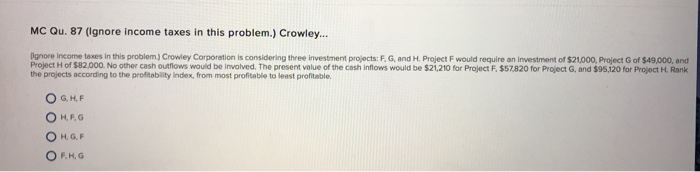# Question & Answer: Crowley Corporation is considering three investment projects: F, G, and H. Project F would require an investment of \$21000, Project G of…..Crowley Corporation is considering three investment projects: F, G, and H. Project F would require an investment of \$21000, Project G of \$49,000, and Project H of \$82,000. No other cash outflows would be involved. The present value of the cash inflows would be \$21,210 for Project F.\$57820 for Project G, and \$95,120 for Project H. Rank the projects according to the profitability index, from most profitable to least profitable. G, H, F H, F, G H, G, F F, H, G

Profitability Index = Present Value of Cash Inflows / Present Value of Cash Outflows

Don't use plagiarized sources. Get Your Custom Essay on
Question & Answer: Crowley Corporation is considering three investment projects: F, G, and H. Project F would require an investment of \$21000, Project G of…..
GET AN ESSAY WRITTEN FOR YOU FROM AS LOW AS \$13/PAGE

Project F:

Profitability Index = \$ 21,210 / \$ 21,000

= 1.01

Project G:

Profitability Index = 57,820/ 49,000

= 1.18

Project H:

Profitability Index = 95,120 / 82,000

= 1.16

Hence, Ranking done on basis of most profitable to least profitable (i.e. Highest Profitability Index to Lowest Profitability Index)

G , H ,F is the correct answer